## Example Questions

### Example Question #17 : Percentage

Quantitative Comparison

Quantity A: 10% of $45 Quantity B: 45% of$10

Quantity B is greater.

Quantity A is greater.

The relationship cannot be determined from the information given.

The two quantities are equal.

The two quantities are equal.

Explanation:

Quantity A: .1 * 45 = $4.50 Quantity B: .45 * 10 =$4.50

Therefore the two quantities are equal.  This is always true: a% of $b = b% of$a.

### Example Question #18 : Percentage

What percent of 5 is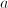?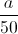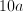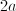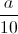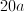Explanation: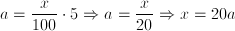### Example Question #1 : Whole And Part

Quantity A:, whereis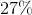ofQuantity B:, whereis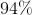ofWhich of the following is true?

Quantity B is larger.

Quantity A is larger.

A comparison cannot be detemined from the given information.

The two quantities are equal.

Quantity B is larger.

Explanation:

This type of problem is very easy.  You merely need to translate the text into the form of an equation.  For this, remember that "of" is translated as multiplication and "is" as equality.  This gives us the following.

Quantity A:isofBecomes...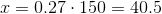Quantity B:isofBecomes...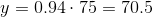Therefore, quantity B is larger.

### Example Question #1 : How To Find The Part From The Whole

Quantity A:, whereis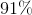of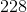Quantity B:, whereis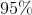of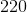Which of the following is true?

Quantity B is larger.

Quantity A is larger.

The two quantities are equal.

A comparison cannot be detemined from the given information.

Quantity B is larger.

Explanation:

This type of problem is very easy.  You merely need to translate the text into the form of an equation.  For this, remember that "of" is translated as multiplication and "is" as equality.  This gives us the following.

Quantity A:isofBecomes...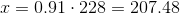Quantity B:isofBecomes...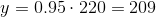Therefore, quantity B is larger.

### Example Question #2 : How To Find The Part From The Whole

Quantity A:, whereis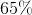of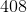Quantity B:, whereisof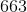Which of the following is true?

The two quantities are equal.

Quantity B is greater.

Quantity A is greater.

A comparison cannot be detemined from the given information.

The two quantities are equal.

Explanation:

This type of problem is very easy.  You merely need to translate the text into the form of an equation.  For this, remember that "of" is translated as multiplication and "is" as equality.  This gives us the following.

Quantity A:isofBecomes...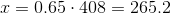Quantity B:isofBecomes...Therefore, the two quantities are equal.

### Example Question #22 : Percentage

A bag contains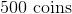.are quarters,are dimes and the rest are nickels. How much money is in the bag in nickels?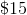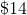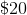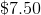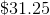To solve this problem we must first find what percent of the money in the bag is in nickels. We know that combined, quarters and  dimes make up 40% of the coins and that the rest are nickels. Therefore 60% of the money in the bag are nickels. We then multiply the total amount of coins in the bag with that percentage in order to find out how many nickels are in the bag.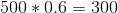.  There are 300 nickels in the bag and nickels are worth 5 cents each. Therefore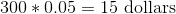worth of nickels in the bag.